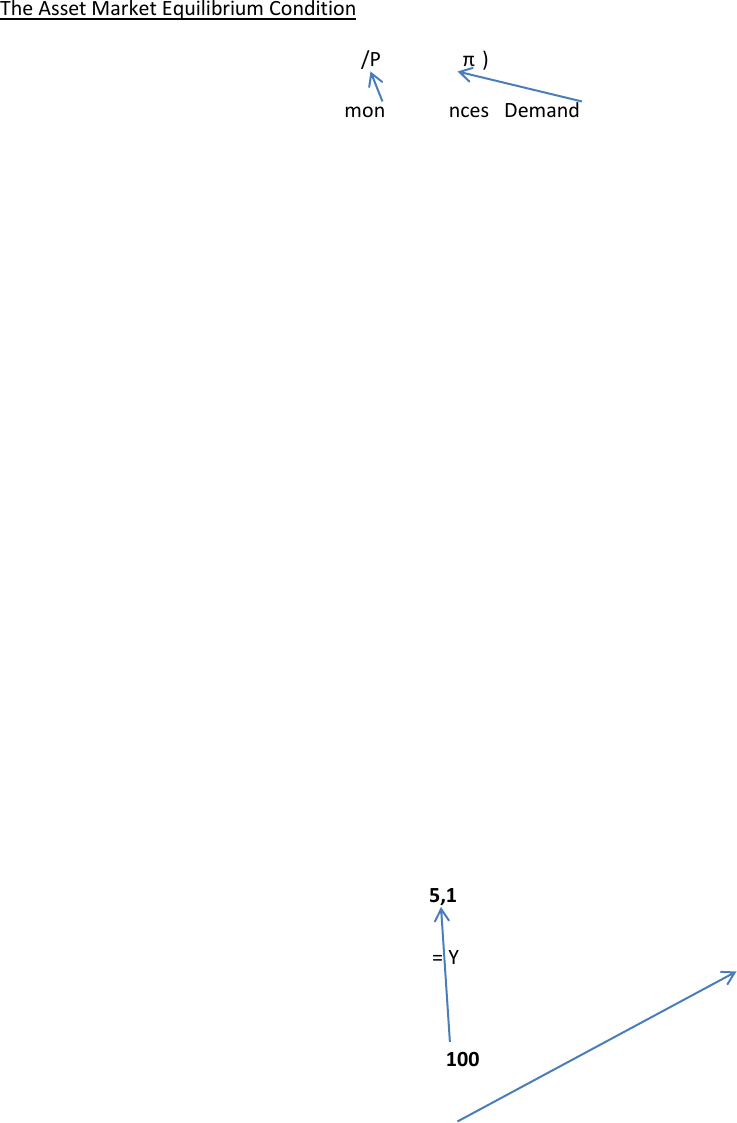# Economics 2152A/B Lecture Notes - Economic Equilibrium, Money Supply

16 views2 pages
Page:
of 2The Asset Market Equilibrium Condition
- Equilibrium in money market, M/P = L(Y,r + πe)
Real money balances Demand for real money
A) M is determined by the central bank
B) Labour market determines the level of employment , which in turn determines Y
C) Given Y and πe (fixed for now) the goods market condition determines r
With all the variables determined in a b and c the asset market equilibrium condition determines the
price level
To illustrate the role of price level,
P = M/ L(Y, r + πe) ie. The price level is the ratio of the nomial money supply to real
money demand
P/P = inflation =
M/M = rate of change in money supply -
L(r + πe)/ L(r + πe) = rate of change in real money demand
Pg. 254 from textbook #1
Md/P = 5000 + 0.2Y 1000i
I = interest paid on non-monetary assets
Im = 0 (interest paid on money)
P = 100, Y = 1000, I = 10%
A) Md/P = 5000 + 0.2(1000) 1000(0.10) = 5,100
B) M = Md/100 = 5,100 Md = 510,000
C) V = Nominal GPD/Nominal money stock = Y.P/Md = 1000(100)/510,000 = 0.196
P = 200 now
A) Md/P = 5000 + 0.2(1000) 1000(0.10) = 5,100
B) M = Md/200 = 1,020,000
C) V = Y.P/Md = 1000(200)/1,020,000 = 0.196
As Y goes up, V goes up
As I goes up, V goes up
V = Y/Md/P
V = Y
5000 + 0.2Y 1000i
Chapter 5 Saving and Investment in Open Economy
Balance of Payment:
- Current Account (CA)
- Capital Account physical capital, financial capital (KA)
- Official Settlements
- CA + KA = 0
Net foreign assets & the balance of payment accounts:
Net foreign assets: are a country’s foreign assets minus its foreign liabilities (domestic assets which
belong to foreigners)
Net foreign assets may change in value due to:
- Prices of assets may change
- Acquisition of new assets by domestic company or foreigners
CA surplus = KA deficit = net acquisition of foreign assets = net foreign lending = net export (if NFP & net
transfers are 0)
Goods market in an open economy
S = I + CA
Sd = Id + CA
= Id + (NX + NFP)
A small open economy is small enough to be able to determine the rate of interest internationally;
rather it is interest rate taker in the global market.